# Hack 36. Calculate the Distance Between GPS Locations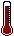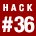If you have GPS coordinates you can calculate the distance between two points.

GPS coordinates are latitude, longitude, and altitude values. With a little trigonometry you can calculate the "crow flies" distance between two pairs of ordinates.

When the two points are close together (no more than a few hundred miles) you can approximate by treating the Earth's surface as flat rather than curved. A single degree of latitude translates to about 111 km (69 miles) everywhere on the planet. That is 6378p / 180° km. The radius of the Earth is 6378 km or 3,963 miles.

A degree of longitude varies. At the equator one degree of longitude is worth the same as one degree of latitude, but at higher latitudes the lines get closer together: in Edinburgh Scotland, at a latitude of 56°, a single degree of longitude is only 62 km. That is (6378km)p(cos(56°)) / 180°.

Suppose you have some GPS coordinates in a table, as shown in Table 5-17.

Table 5-17. The gps table

name North (latitude) East (longitude)
home 55.954742 3.207630
napier 55.932809 3.214617
zzuli 34.749660 113.670094

You need to turn these values into radians before you can apply the trig functions. You are also going to need the radius of the Earth so that you can put that in as a constant:

```CREATE VIEW gpsRad AS
SELECT name, 3.14159265359*e/180 AS lon
, 3.14159265359*n/180 AS lat
, 6378 AS R
FROM gps```

Work (napier) is dx kilometers east of Home and dy kilometers to the north in this query:

```mysql> SELECT p1.R*(p2.lon-p1.lon)*COS(p1.lat) AS dx,
-> p1.R*(p2.lat-p1.lat) AS dy
-> ON (p1.name='home' AND p2.name='napier');
+-------------------+------------------+
| dx | dy |
+-------------------+------------------+
| -0.43544683467495 | -2.4417242388667 |
+-------------------+------------------+```

To get the actual distance in kilometers you need to apply the Pythagorean theorem, as shown in the following code snippet and illustrated in Figure 5-3:

```mysql> SELECT SQRT(dx*dx+dy*dy) FROM
-> (SELECT p1.R*(p2.lon-p1.lon)*COS(p1.lat) AS dx,
-> p1.R*(p2.lat-p1.lat) AS dy
-> ON (p1.name='home' AND p2.name='napier')) t;
+-------------------+
| SQRT(dx*dx+dy*dy) |
+-------------------+
| 2.4802482142918 |
+-------------------+ ```

Figure 5-3. Calculating the distance from dx and dy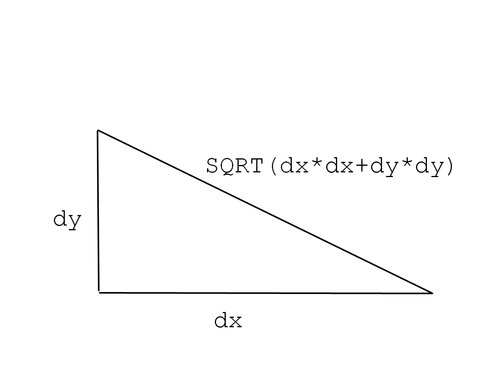5.13.1. Hacking the Hack

If you want to measure bigger distances you need to do the 3D geometry properly. Using the preceding method to measure the distance between the ZhengZhou University of Light Industry in China and Napier University in the UK gives:

```mysql> SELECT SQRT(dx*dx+dy*dy) FROM
-> (SELECT p1.R*(p2.lon-p1.lon)*COS(p1.lat) AS dx,
-> p1.R*(p2.lat-p1.lat) AS dy
-> ON (p1.name='zzuli' AND p2.name='napier')) t;
+-------------------+
| SQRT(dx*dx+dy*dy) |
+-------------------+
| 10947.852030902 |
+-------------------+```

You know that there is a problem because if you estimate the distance from the UK to China by swapping the start and end points, it comes out as:

```mysql> SELECT SQRT(dx*dx+dy*dy) FROM
-> (SELECT p1.R*(p2.lon-p1.lon)*COS(p1.lat) AS dx,
-> p1.R*(p2.lat-p1.lat) AS dy
-> ON (p1.name='napier' AND p2.name='zzuli')) t;
+-------------------+
| SQRT(dx*dx+dy*dy) |
+-------------------+
| 7660.533655487 |
+-------------------+```

The GPS ordinates are the lon and lat angles in Figure 5-4. You can work out the (x,y,z) coordinates, relative to the center of the Earth. With two triples of x, y, z you can apply the Pythagorean theorem, which will give you the straight line distance:

x = R cos(lat) cos(lon)

y = R cos(lat) sin(lon)

z = R sin(lat)

Figure 5-4. (x,y z) coordinates from latitude and longitude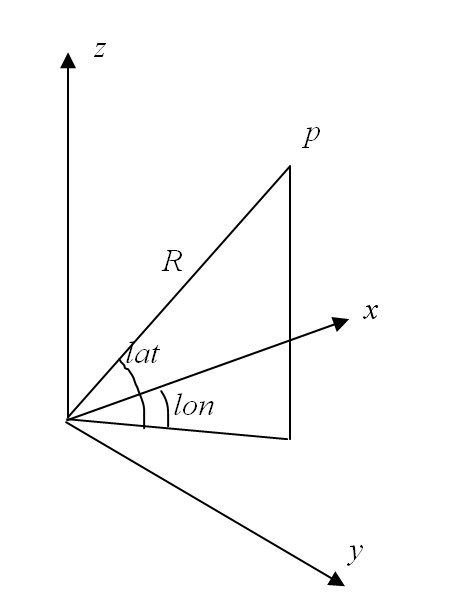The gpsTrig view does the trigonometry calculations based on the gpsRad view from before:

```CREATE VIEW gpsTrig AS
SELECT name, R, COS(lat) AS cos_lat, SIN(lat) AS sin_lat,
COS(lon) AS cos_lon, SIN(lon) AS sin_lon

Now you can calculate the x, y, and z coordinates relative to the center of the Earth:

```CREATE VIEW gpsGlb AS
SELECT name, R*cos_lat*cos_lon AS x,
R*cos_lat*sin_lon AS y,
R*sin_lat AS z
FROM gpsTrig;```

You also can find the vector between two points in three dimensions:

```mysql> SELECT p1.x-p2.x AS dx, p1.y-p2.y AS dy, p1.z-p2.z AS dz
-> FROM gpsGlb p1 JOIN gpsGlb p2
-> ON (p1.name='zzuli' AND p2.name='napier');
+------------------+-----------------+------------------+
| dx | dy | dz |
+------------------+-----------------+------------------+
| -5671.0949161728 | 5000.0390465225 | -1648.0329880006 |
+------------------+-----------------+------------------+```

To find the distance in kilometers you must apply the Pythagorean theorem:

```mysql> SELECT SQRT(dx*dx+dy*dy+dz*dz) FROM
-> (SELECT p1.x-p2.x AS dx, p1.y-p2.y AS dy, p1.z-p2.z AS dz
-> FROM gpsGlb p1 JOIN gpsGlb p2
-> ON (p1.name='zzuli' AND p2.name='napier')) t;
+-------------------------+
| SQRT(dx*dx+dy*dy+dz*dz) |
+-------------------------+
| 7738.0695748054 |
+-------------------------+```

Of course, this is the straight line distancein the worst case, this route will take you right through Earth's molten core. It's probably better to go around the outside, and you can make that adjustment with just a little more circle geometry, as shown in Figure 5-5.

Figure 5-5. Calculating the length of an arc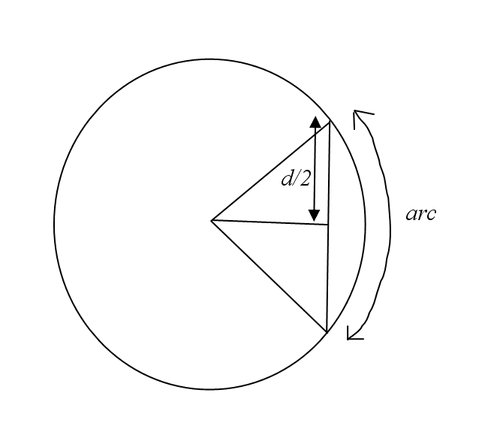To get the length of the arc where the straight line distance is d you can use 2*R*asin(d/2/R), where R is the radius of the circle:

```mysql> SELECT 2*R*ASIN(d/2/R) AS arc FROM
-> (SELECT 6378 AS R) t0,
-> (SELECT SQRT(dx*dx+dy*dy+dz*dz) AS d FROM
-> (SELECT p1.x-p2.x AS dx, p1.y-p2.y AS dy, p1.z-p2.z AS dz
-> FROM gpsGlb p1 JOIN gpsGlb p2
-> ON (p1.name='zzuli' AND p2.name='napier')
-> ) t1) t2;
+-----------------+
| arc |
+-----------------+
| 8314.4181316969 |
+-----------------+```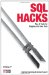SQL Hacks
ISBN: 0596527993
EAN: 2147483647
Year: 2004
Pages: 147